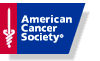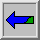American Cancer Society Please feel free to forward your comments, suggestions, and corrections to Dr. James L. Kepner

# Sample Size Calculator: One-Sample Designs

### Background

This program determines the sample size necessary to attain a specified size and power for an exact test of one proportion. The hypotheses to be tested are of the form:

H0: p=p0
H1: p>p0
The user must submit a value for p0, an upper bound for alpha (i.e., the probability of committing a Type I error), and a lower bound for the power at the point p = p0+delta,   where 0 < delta < (1-p0).

Upon taking these study constraints into consideration, the calculator will then recommend a design that is optimal by the following criteria:
1. The first stage sample size, n1, is as small as possible.
2. The second stage sample size (if a two-stage design is requested), n2, is as small as possible and satisfies n2 <= n1.
3. The third stage sample size, (if a three-stage design is requested), n3, is as small as possible and satisfies n3 <= n2.
4. The actual significance level, or size, of the test satisfies size <= alpha, where alpha is the significance level specified by the user.
5. The actual power at p = p0+delta, apower, satisfies apower >= power, where power is the user-specified minimum acceptable power.

### Step One

Enter the following information from your study*:

 Alpha Power p0 Delta

### Step Two

Select type of study:

### Step Three

Will this calculation take a significant amount of time?
No, Display Results
Yes, E-Mail Results